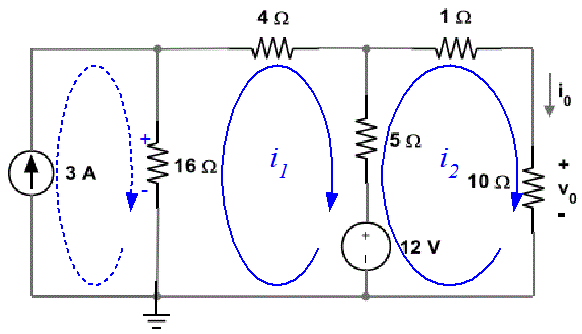# Mesh current analysis

## Homework Statement

Solve using the Mesh-current method. Determine the current magnitudes and the voltages across all elements

i=v/r
i1+i2+i3..=0

## The Attempt at a Solution

loop 1:
16(i3-3)+25i1-5i2=12
loop2:
4i1-5i2=15

1) i1=5/9i2-1

substituting 1) into loop2 gives
-25/9i2+5+16i2=-12
i2=1.1344

substituting i2=1.1233 into 1) gives i1=4.672

Can someone show me where I'm going wrong? I think I messed up the signs somewhere, I tried it a few ways and can't get it to satisfy KCL after I check my answer.

#### Attachments

•node.png
7 KB · Views: 352

Hesch
Gold Member
Can someone show me where I'm going wrong? I think I messed up the signs somewhere
That's impossible, because you have not sketched any loops or any currents ( except for i0 ).

You are the one to decide positive directions, etc.

My i1 is the middle loop (clockwise direction) and my i2 is the right loop (counter clockwise direction)
My i3 is the far left loop (clockwise direction) and the current was given as 3A

gneill
Mentor
My i1 is the middle loop (clockwise direction) and my i2 is the right loop (counter clockwise direction)
My i3 is the far left loop (clockwise direction) and the current was given as 3A

loop 1:
16(i3-3)+25i1-5i2=12
loop2:
4i1-5i2=15

Given the above I don't see how you arrived at your two loop equations. If i3 has a given value, why does the i3 variable appear in the Loop1 equation? Loop 2 has a total of 16 Ohms in it, yet I don't see where your equation accounts for i2 flowing through that, nor how i1 is flowing through 4 Ohms for that loop when I see that a 5 Ohm resistor borders the loops.

I think you need to redo your loop equations. In general it's usually beneficial to choose the same loop direction for all loops (one reason has to do with some really handy and time saving matrix solving methods that you'll learn about soon) Here's a diagram to help:I suggest that as a first step you might eliminate the 3 A mesh by moving its effect into the i1 loop as a fixed voltage source (due to its flowing through the 16 Ohm resistor).

Hesch
Gold Member
@orangeincup
I suggest that as a first step you might eliminate the 3 A mesh by moving its effect into the i1 loop as a fixed voltage source
Your i3 = 3A, simply because i3 is the only loop-current that passes the current-source.

As for i2-loop you will get ( according to figure in #4 ):

+12V - ( 5 + 1 + 10 )Ω * i2 - 5Ω( -i1 ) = 0 ( i1 has opposite direction as to i2 )

And the equation as for i1?

Now, solve the 3 equations ( your calculator can probably do that simultaniously), or substitute i3 = 3A ( leaving 2 equations ).

i16Ω upward = i1 - i3 and so on.

Calculate the voltages by ohm's law.